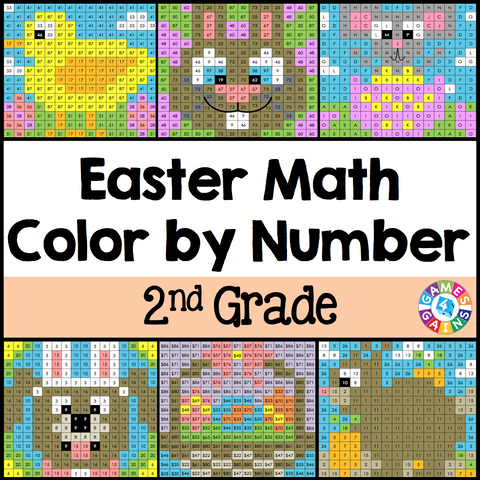Easter Math Color-by-Number - 2nd Grade

• \$480

Easter Math Color-by-Number set comes with 6 Easter Math color-by-number activities for reviewing 2nd grade math skills. This Easter Math math set is perfect to use in centers, in small groups, or with the whole class!

Here are the skills covered by these Easter Math math color-by-number activities:

• Determining the unknown in addition and subtraction sentences within 100
• Solving word problems involving lengths
• Calculating the value of a group of twenty, ten, five, and one dollar bills
• Solving problems using information presented in bar graphs
• Describing shapes when partitioned into halves, thirds, or fourths
• Drawing and finding the total number of objects in rectangular arrays

Included with this Easter Math Math Color-by-Number set:
Instructions
6 color by number worksheets
6 student problem worksheets